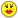+0

# How do you find the area and perimeter of a figure by only using points?

0
598
3

How do you find the area and perimeter of a figure by only using points?

For example:

(-5,3) (-1,2) (0,6)

Sep 9, 2014

#2
+5

I. How do you find the area of a figure by only using points?

For n points, the points must be oriented:

$$\boxed{2A= \sum \limits_{i=1}^n(y_i+y_{i+1})(x_i-x_{i+1}) \quad x_{n+1} = x_1 \quad y_{n+1} = y_1}$$ [Carl Friedrich Gauß]

For example (-5,3) (-1,2) (0,6), the points are oriented!

$$\begin{array}{|c|r|r|} \hline Point & x & y \\ \hline 1 &-5&3 \\ 2 &-1&2 \\ 3 &0&6 \\ \hline \end{array}$$

$$\begin{array}{rcl} 2A & = & (y_1+y_2)(x_1-x_2)+(y_2+y_3)(x_2-x_3)+(y_3+y_1)(x_3-x_1) \\ 2A & = & (3+2)(-5+1)+(2+6)(-1-0)+(6+3)(0+5)\\ 2A & = & (5)(-4)+(8)(-1)+(9)(5)\\ 2A & = & -20 - 8 + 45\\ 2A & = & 17\\ A & = & 8.5 \end{array}$$

II. How do you find the Perimeter of a figure by only using points?

$$\boxed{Perimeter = \sum \limits_{i=1}^n \sqrt{(x_{i+1}-x_i)^2+(y_{i+1}-y_i)^2}\quad x_{n+1} = x_1 \quad y_{n+1} = y_1} }$$

Example:

$$\begin{array}{|c|r|r|} \hline Point & x & y \\ \hline 1 &-5&3 \\ 2 &-1&2 \\ 3 &0&6 \\ \hline \end{array}$$

$$\begin{array}{rcl} Perimeter & = & \sqrt{(x_2-x_1)^2+(y_2-y_1)^2}+ \sqrt{(x_3-x_2)^2+(y_3-y_2)^2}+ \sqrt{(x_1-x_3)^2+(y_1-y_3)^2} \\ & = & \sqrt{(-1+5)^2+(2-3)^2}+ \sqrt{(0+1)^2+(6-2)^2}+ \sqrt{(-5-0)^2+(3-6)^2} \\ & = & \sqrt{(4)^2+(-1)^2}+ \sqrt{(1)^2+(4)^2}+ \sqrt{(-5)^2+(-3)^2} \\ & = & \sqrt{16+1}+ \sqrt{1+16}+ \sqrt{25+9} \\ & = & \sqrt{17}+ \sqrt{17}+ \sqrt{34} \\ Perimeter & = & = 14.0771631461 \end{array}$$.
Sep 10, 2014

#1
+5

Find the distance between all three points using the distance formula.....

Add all these results for the perimeter

To find the area of this triangle  ....let's use something called the "semi-perimeter"   = S  ...this is given by .....(A + B + C) / 2    where A, B and C are the side lengths

Then the area is given by....

√[S * (S - A) * (S - B) * (S - C)]

If you get stuck, let me know..........!!!Sep 9, 2014
#2
+5

I. How do you find the area of a figure by only using points?

For n points, the points must be oriented:

$$\boxed{2A= \sum \limits_{i=1}^n(y_i+y_{i+1})(x_i-x_{i+1}) \quad x_{n+1} = x_1 \quad y_{n+1} = y_1}$$ [Carl Friedrich Gauß]

For example (-5,3) (-1,2) (0,6), the points are oriented!

$$\begin{array}{|c|r|r|} \hline Point & x & y \\ \hline 1 &-5&3 \\ 2 &-1&2 \\ 3 &0&6 \\ \hline \end{array}$$

$$\begin{array}{rcl} 2A & = & (y_1+y_2)(x_1-x_2)+(y_2+y_3)(x_2-x_3)+(y_3+y_1)(x_3-x_1) \\ 2A & = & (3+2)(-5+1)+(2+6)(-1-0)+(6+3)(0+5)\\ 2A & = & (5)(-4)+(8)(-1)+(9)(5)\\ 2A & = & -20 - 8 + 45\\ 2A & = & 17\\ A & = & 8.5 \end{array}$$

II. How do you find the Perimeter of a figure by only using points?

$$\boxed{Perimeter = \sum \limits_{i=1}^n \sqrt{(x_{i+1}-x_i)^2+(y_{i+1}-y_i)^2}\quad x_{n+1} = x_1 \quad y_{n+1} = y_1} }$$

Example:

$$\begin{array}{|c|r|r|} \hline Point & x & y \\ \hline 1 &-5&3 \\ 2 &-1&2 \\ 3 &0&6 \\ \hline \end{array}$$

$$\begin{array}{rcl} Perimeter & = & \sqrt{(x_2-x_1)^2+(y_2-y_1)^2}+ \sqrt{(x_3-x_2)^2+(y_3-y_2)^2}+ \sqrt{(x_1-x_3)^2+(y_1-y_3)^2} \\ & = & \sqrt{(-1+5)^2+(2-3)^2}+ \sqrt{(0+1)^2+(6-2)^2}+ \sqrt{(-5-0)^2+(3-6)^2} \\ & = & \sqrt{(4)^2+(-1)^2}+ \sqrt{(1)^2+(4)^2}+ \sqrt{(-5)^2+(-3)^2} \\ & = & \sqrt{16+1}+ \sqrt{1+16}+ \sqrt{25+9} \\ & = & \sqrt{17}+ \sqrt{17}+ \sqrt{34} \\ Perimeter & = & = 14.0771631461 \end{array}$$heureka Sep 10, 2014
#3
0

I don't remember seeing that  area formula before Heureka.  I've got a lot to learn.Your dispay is as brilliant as we have all come to expect.  Thanks.  :)

You know Chris I have seen you use that formula a number of times and it still has not sunk into my brain!Thanks( I think I may reserve that icon for when i am feeling more "blond" than usual)

Sep 10, 2014